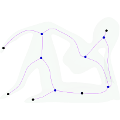CGAL 4.8.2 - Triangulated Surface Mesh Skeletonization
Triangulated Surface Mesh Skeletonization ReferenceXiang Gao, Sébastien Loriot and Andrea Tagliasacchi
This package provides a (1D) curve skeleton extraction algorithm for a triangulated polygonal mesh without borders based on the mean curvature flow. The particularity of this skeleton is that it captures the topology of the input. For each skeleton vertex one can obtain its location and its corresponding vertices from the input mesh. The code is generic and works with any model of the FaceListGraph concept.

Introduced in: CGAL 4.7
Depends on: CGAL and Solvers
BibTeX: cgal:glt-tsms-16b
Windows Demo: See Polyhedral Surface
Common Demo Dlls: dlls

## Concepts

• MeanCurvatureSkeletonizationTraits
• NormalEquationSparseLinearAlgebraTraits_d

## Classes

• CGAL::Mean_curvature_flow_skeletonization

Concepts

## Classes

class  CGAL::Mean_curvature_flow_skeletonization< TriangleMesh, Traits_, VertexPointMap_, SolverTraits_ >
Function object that enables to extract the mean curvature flow skeleton of a triangulated surface mesh. More...

## Functions

template<class TriangleMesh >
void CGAL::extract_mean_curvature_flow_skeleton (const TriangleMesh &tmesh, typename Mean_curvature_flow_skeletonization< TriangleMesh >::Skeleton &skeleton)
extracts a medially centered curve skeleton for the triangle mesh tmesh. This function uses the class CGAL::Mean_curvature_flow_skeletonization with the default parameters. This function is provided only if Eigen 3.2 (or greater) is available and CGAL_EIGEN3_ENABLED is defined. More...

## Function Documentation

template<class TriangleMesh >
 void CGAL::extract_mean_curvature_flow_skeleton ( const TriangleMesh & tmesh, typename Mean_curvature_flow_skeletonization< TriangleMesh >::Skeleton & skeleton )

extracts a medially centered curve skeleton for the triangle mesh tmesh. This function uses the class CGAL::Mean_curvature_flow_skeletonization with the default parameters. This function is provided only if Eigen 3.2 (or greater) is available and CGAL_EIGEN3_ENABLED is defined.

Precondition
tmesh is a triangle mesh without borders and having exactly one connected component.
The specialization boost::property_map<TriangleMesh, CGAL::vertex_point_t>::type and get(vertex_point, tmesh) are defined.
The value type of boost::property_map<TriangleMesh, CGAL::vertex_point_t>::type is a point type from a CGAL Kernel.
Template Parameters
 TriangleMesh a model of FaceListGraph
Parameters
 tmesh input mesh skeleton graph that will contain the skeleton of tmesh. For each vertex descriptor vd of skeleton, the corresponding point and the set of input vertices that contracted to vd can be retrieved using skeleton[vd].point and skeleton[vd].vertices respectively.

#include <CGAL/extract_mean_curvature_flow_skeleton.h>

Examples:
Surface_mesh_skeletonization/segmentation_example.cpp, Surface_mesh_skeletonization/simple_mcfskel_example.cpp, and Surface_mesh_skeletonization/simple_mcfskel_sm_example.cpp.